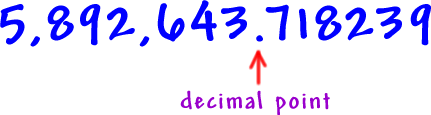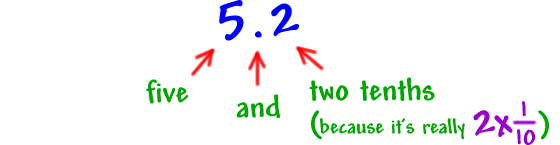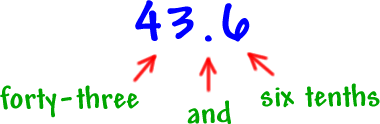# Decimals

Decimal numbers are made up of a whole number part, a decimal (or fraction) part, and the decimal point.

The place values to the right of the decimal point of the number shown above are given below:The proper way to read a decimal number is to (1) read the whole number part, (2) read the decimal point as the word "and", and (3) read the decimal part.

Here are three examples:

Example 1:Example 2:Example 3:What is the correct way to read the following number?
63.174

Select the number that matches the word:
seven and twenty-nine ten-thousandths